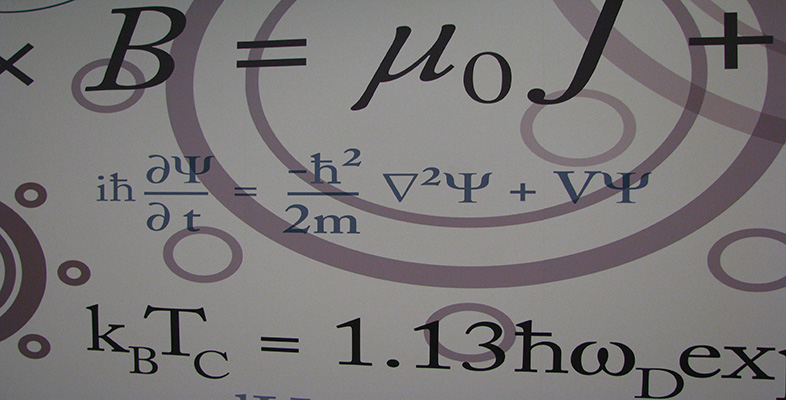Number systems

This free course is available to start right now. Review the full course description and key learning outcomes and create an account and enrol if you want a free statement of participation.

Free course

2.10 The complex exponential function

Consider the real exponential function f (x) = ex (that is, f (x) = exp x). We now extend the definition of this function to define a function f(z) = ez whose domain and codomain are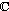.

We expect complex powers of e to satisfy the familiar properties of real powers of e. For example, we expect that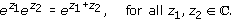If this is to be achieved, then the definition of ez has to be as follows.

Definition

If z = x + iy, then ez = exeiy = ex(cos y + i sin y).

Example 8

Show that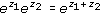for all complex numbers z1 and z2.

Solution

We use Strategy 1.

Suppose that z1 = x1 + iy1 and z2 = x2 + iy2. Then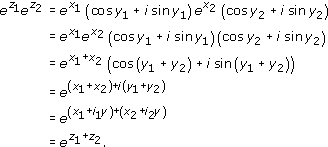We assume that if x1, x2 are real numbers, then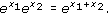Exercise 26

• (a)  Using the above definition for ez and de Moivre's Theorem, show that

•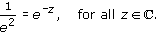• (b)  Show that ez1/ ez2 = ez1z2, for all z1, z2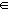.

Solution

• (a)  Let z = x + iy; then

•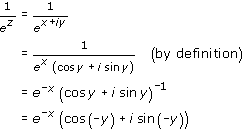• (by de Moivre's Theorem with n = − 1)

•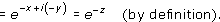• (b)

•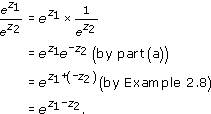So the rules for multiplication and division of complex powers of e are exactly the same as those for real powers. Furthermore, when the exponent z is real, that is when z = x + 0i, where x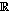, the definitions of a real and a complex power of e coincide, since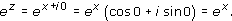On the other hand, if z = 0 + iy, where y, then the definition gives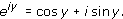This is known as Euler's formula. Putting y =, we obtain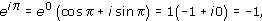or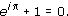This is a remarkable relationship between five important numbers: 0, 1, i,and e.

The formula eiy = cos y + i sin y gives us an alternative form for the expression of a complex number in polar form. If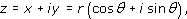then we can write cos θ + i sin θ as eiθ, so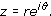Some texts refer to reiθ as polar form.

A complex number expressed in this way is said to be in exponential form. Using this notation, de Moivre's Theorem becomes the simple result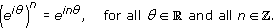We can use the complex exponential function to find some further useful trigonometric identities. At the end of Section 2.9 we showed how de Moivre's Theorem can be used to express the sine or cosine of a multiple of θ in terms of powers of sin θ and cos θ. Here we do the opposite, expressing a power of sin θ or cos θ as a combination of sines or cosines of multiples of θ.

First, we deduce two useful equations. We know that, for all θ,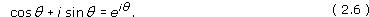Also, since cos(−θ) + i sin(−θ)=cos θ i sin θ, we have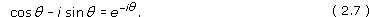Adding equations 2.6 and 2.7 gives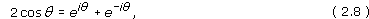and subtracting them gives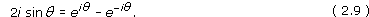Equations 2.8 and 2.9 enable us to express cos θ and sin θ as combinations of complex exponentials, and it is these equations we use to obtain the identities we are looking for.

Example 9

• (a)  Show that

•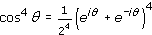.

• (b)  Expand the expression (eiθ + eiθ)4 using the Binomial Theorem, and hence show that

•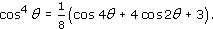Solution

• (a)  2cos θ = eiθ + eiθ, so (2 cos θ)4 = (eiθ + eiθ)4.

• Hence

•.

• (b)

•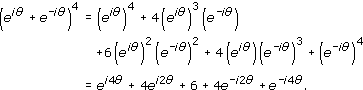• Hence

•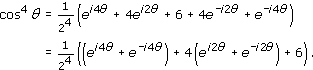• Using Equation 2.8 first with 4θ in place of θ and then with 2θ in place of θ, we have

•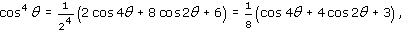• as required.

Exercise 27

• (a)  Show that

•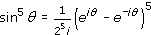.

• (b)  Expand (eiθeiθ)5 using the Binomial Theorem, and hence show that

•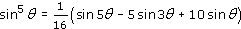.

Solution

• (a)  2i sin θ = eiθe, so (2i sin θ)5 =(ei θei θ)5.

• Hence

•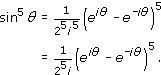• (b)

•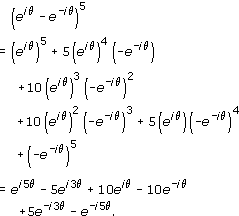• Hence

•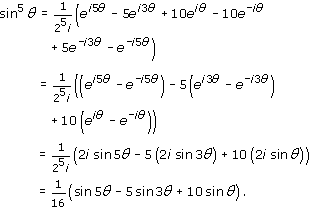M208_6